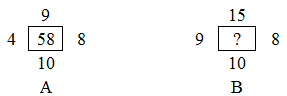Question 9

# Assuming that the numbers in each of the following figures follow a similar pattern, select the option that can replace the question mark (?) in figure B.Solution

We get answer after Multiplication of Top and bottom , similarly multiply left and right number then Result of multiplication subtracted from each othr

$$9\times10-4\times8=58$$

$$15\times10-9\times8=78$$